## Friday, November 29, 2019

### Major updates to phylosig function, including a new plotting method

The function phylosig of phytools must be one of the most frequently used functions in the package, with more than 400 different articles specifically referencing 'phytools' & 'phylosig' (according to Google Scholar, as of 29 Nov. 2019).

As such, and even though it dates to the early days of phytools, I have tried to leave the function relatively untouched. Whilst working on an R project today (to be announced later) I finally caved & pushed a whole bunch of updates to the function - including totally new print and plot methods and a number of other changes. In so doing, however, I made a significant effort to ensure that the function would still remain compatible with any scripts that had been written around its prior output format.

The following is a demo of some of the new functionality of phylosig. It uses an old dataset for body mass & home range size in mammals from Garland et al. (1992). I have posted both files online here: mammalHR.phy & mammalHR.csv.

library(phytools)
packageVersion("phytools")

##  '0.7.7'

mammal.tree<-read.tree("mammalHR.phy")
plotTree(mammal.tree,fsize=0.8,ftype="i",
lwd=1)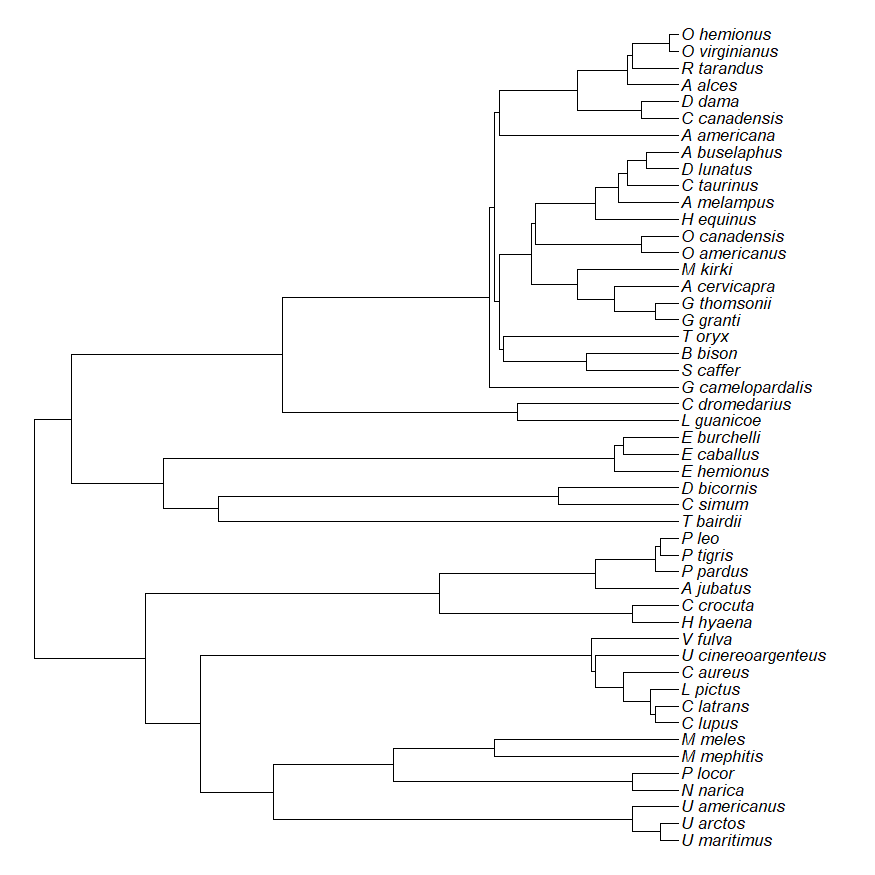Now we can proceed to read in our data too:

mammal.data<-read.csv("mammalHR.csv",
row.names=1)
mammal.data

##                    bodyMass homeRange
## U_maritimus          265.00   115.600
## U_arctos             251.30    82.800
## U_americanus          93.40    56.800
## N_narica               4.40     1.050
## P_locor                7.00     1.140
## M_mephitis             2.50     2.500
## M_meles               11.60     0.870
## C_lupus               35.30   202.800
## C_latrans             13.30    45.000
## L_pictus              20.00   160.000
## C_aureus               8.80     9.100
## U_cinereoargenteus     3.70     1.100
## V_fulva                4.80     3.870
## H_hyaena              26.80   152.800
## C_crocuta             52.00    25.000
## A_jubatus             58.80    62.100
## P_pardus              52.40    23.200
## P_tigris             161.00    69.600
## P_leo                155.80   236.000
## T_bairdii            250.00     2.000
## C_simum             2000.00     6.650
## D_bicornis          1200.00    15.600
## E_hemionus           200.00    35.000
## E_caballus           350.00    22.500
## E_burchelli          235.00   165.000
## L_guanicoe            95.00     0.500
## C_dromedarius        550.00   100.000
## G_camelopardalis    1075.00    84.600
## S_caffer            6210.00   138.000
## B_bison              865.00   133.000
## T_oryx               511.00    87.500
## G_granti              62.50    20.000
## G_thomsonii           20.50     5.300
## A_cervicapra          37.50     6.500
## M_kirki                5.00     0.043
## O_americanus         113.50    22.750
## H_equinus            226.50    80.000
## A_melampus            53.25     3.800
## C_taurinus           216.00    75.000
## D_lunatus            130.00     2.200
## A_buselaphus         136.00     5.000
## A_americana           50.00    10.000
## D_dama                55.00     1.300
## A_alces              384.00    16.100
## R_tarandus           100.00    30.000
## O_virginianus         57.00     1.960
## O_hemionus            74.00     2.850

bodyMass<-setNames(log(mammal.data$bodyMass), rownames(mammal.data))  Now, let's compute phylogenetic signal using Blomberg et al.'s K statistic. This is the default method in phylosig: phylosig(mammal.tree,bodyMass)  ## ## Phylogenetic signal K : 0.562551  So far the only change is that it uses a cleaner print method, rather than just spitting back a numeric value. Where it gets a bit more fun is when we use the statistical tests that are implemented in the function. For method="K" this is just a randomization test, as follows: bmassK<-phylosig(mammal.tree, bodyMass,test=TRUE,nsim=10000) bmassK  ## ## Phylogenetic signal K : 0.562551 ## P-value (based on 10000 randomizations) : 1e-04  plot(bmassK)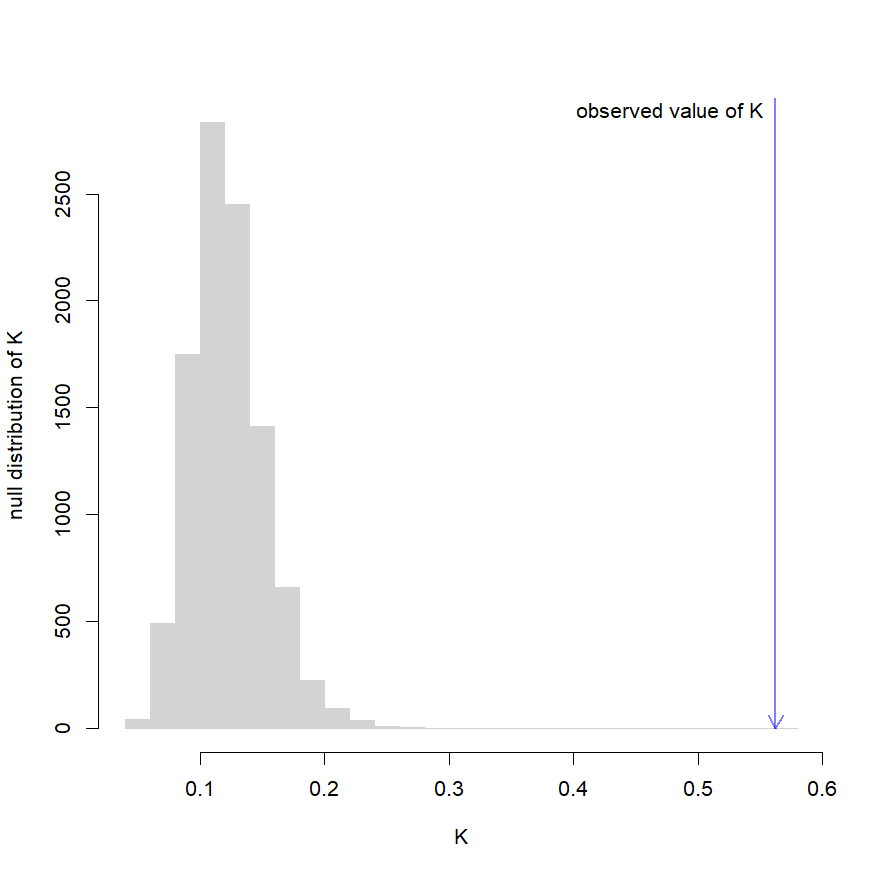That's kind of neat, right? Now let's switch to the other phylogenetic signal metric that's implemented in phylosig: Pagel's $$\lambda$$. phylosig(mammal.tree,bodyMass, method="lambda")  ## ## Phylogenetic signal lambda : 0.99661 ## logL(lambda) : -78.0411  Or, with the plot method: bmassLambda<-phylosig(mammal.tree, bodyMass,method="lambda", test=TRUE) bmassLambda  ## ## Phylogenetic signal lambda : 0.99661 ## logL(lambda) : -78.0411 ## LR(lambda=0) : 35.414 ## P-value (based on LR test) : 2.66558e-09  plot(bmassLambda)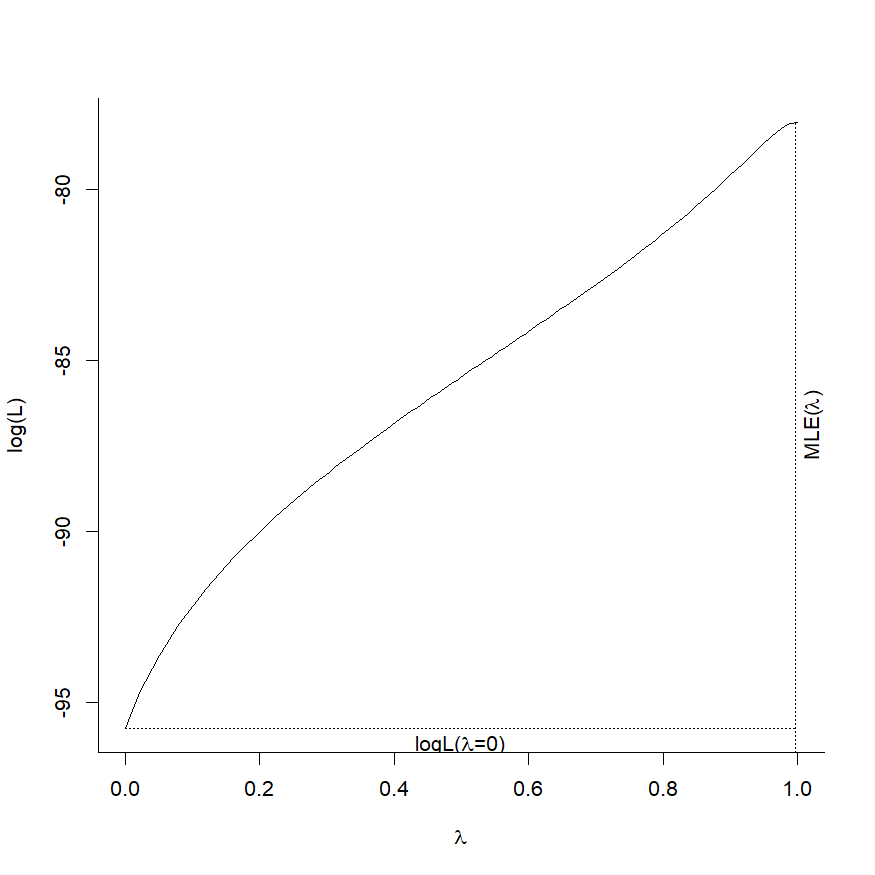Finally, let's switch to analyzing home range size to see if that shows a similar or different pattern: homeRange<-setNames(log(mammal.data$homeRange),
rownames(mammal.data))
hrangeLambda<-phylosig(mammal.tree,
homeRange,method="lambda",
test=TRUE)
plot(hrangeLambda)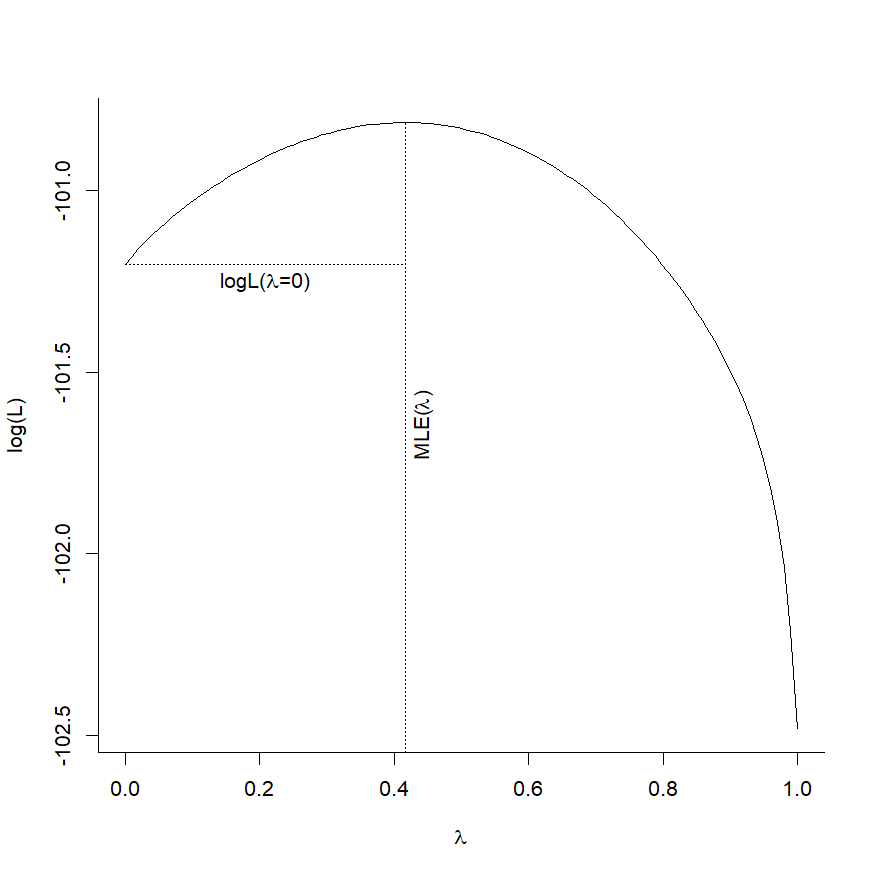Wild.

## Thursday, November 28, 2019

### New function for graphical simulation of Brownian motion on a tree

Here's a new function that creates a two panel plot. The first panel contains a simulated, discrete-time, pure-birth phylogeny of N taxa. The second panel gives the results of a discrete-time Brownian (random walk) simulation on the tree. I developed it for use in teaching, but will add it to phytools shortly:

simBMphylo<-function(n,t,sig2,plot=TRUE,...){
b<-exp((log(n)-log(2))/t)-1
tree<-pbtree(b=b,d=0,t=t,n=n,type="discrete",
tip.label=if(n<=26) LETTERS[n:1] else NULL,
quiet=TRUE)
H<-nodeHeights(tree)
root<-Ntip(tree)+1
xx<-list()
for(i in 1:nrow(tree$edge)){ x<-rnorm(n=tree$edge.length[i],sd=sqrt(sig2))
x<-c(0,cumsum(x))
if(tree$edge[i,1]!=root){ ii<-which(tree$edge[,2]==tree$edge[i,1]) x<-x+xx[[ii]][length(xx[[ii]])] } xx[[i]]<-x } object<-list(tree=tree,x=xx) class(object)<-"simBMphylo" if(plot) plot(object,...) invisible(object) } plot.simBMphylo<-function(x,...){ xx<-x$x
tree<-x$tree H<-nodeHeights(tree) par(mfrow=c(2,1)) plotTree(tree,mar=c(2.1,4.1,3.1,1.1), xlim=c(0,1.05*max(H)),lwd=1) mtext("a)",line=1,adj=0) plot.new() par(mar=c(5.1,4.1,3.1,1.1)) plot.window(xlim=c(0,1.05*max(H)),ylim=range(xx)) axis(1) axis(2) for(i in 1:length(xx)) lines(H[i,1]:H[i,2],xx[[i]]) for(i in 1:Ntip(tree)){ ii<-which(tree$edge[,2]==i)
text(max(H),xx[[ii]][length(xx[[ii]])],
tree$tip.label[i],pos=4,offset=0.4/3) } mtext("b)",line=1,adj=0) title(xlab="time",ylab="phenotype") } print.simBMphylo<-function(x,...){ cat(paste("\nObject of class \"simBMphylo\" with",Ntip(x$tree),"taxa.\n"),
sep="")
cat("To print use plot method.\n\n")
}


Let's see how it works:

library(phytools)
set.seed(30)
simBMphylo(n=6,t=100,sig2=0.01)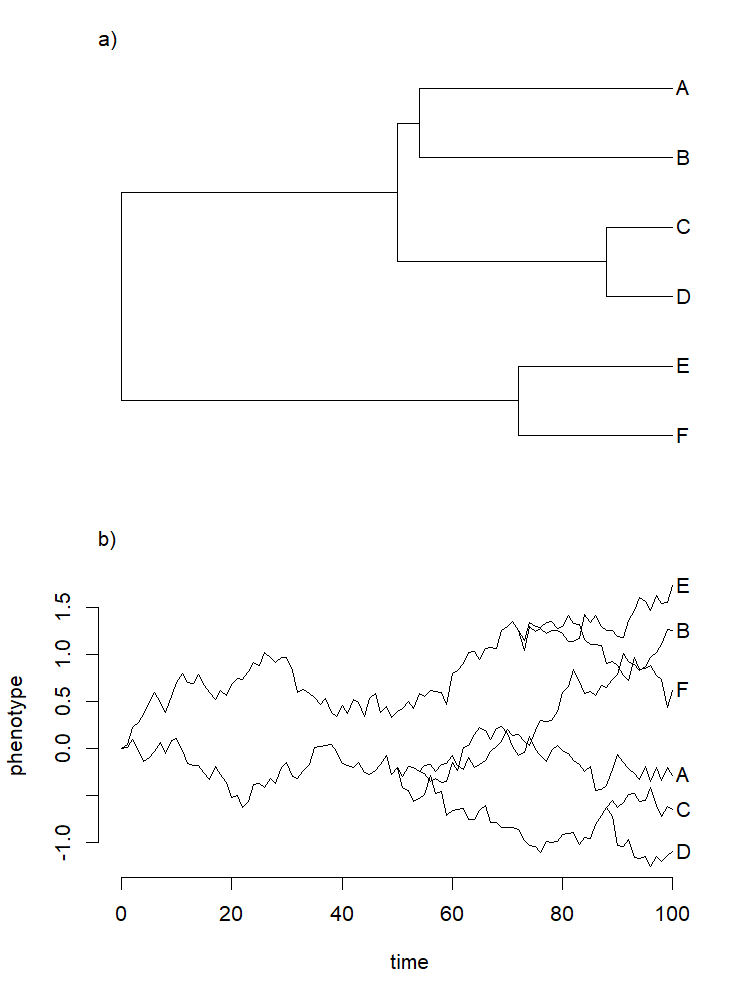That's it. Happy thanksgiving.